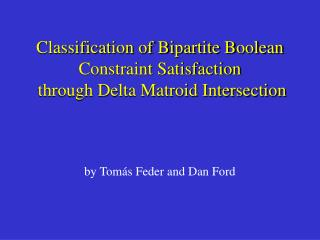# Classification of Bipartite Boolean Constraint Satisfaction through Delta Matroid Intersection - PowerPoint PPT PresentationDownload PresentationClassification of Bipartite Boolean Constraint Satisfaction through Delta Matroid Intersection

Classification of Bipartite Boolean Constraint Satisfaction through Delta Matroid IntersectionDownload Presentation## Classification of Bipartite Boolean Constraint Satisfaction through Delta Matroid Intersection

- - - - - - - - - - - - - - - - - - - - - - - - - - - E N D - - - - - - - - - - - - - - - - - - - - - - - - - - -
##### Presentation Transcript

1. Classification of Bipartite Boolean Constraint Satisfaction through Delta Matroid Intersection by Tomás Feder and Dan Ford

2. Towards a classification with two occurrences per variable 1. If not in Schaefer’s polynomial cases then can simulate all clauses _ _ _ _ xVyVz, xVyVzVt 2. If not delta-matroid then can simulate R = (xy, z): (0, 0, 0) , (1, 1, 1) R (x, y, z) Rxy, z 1. and 2. simulate satisfiability with threeoccurrences per variable NP-complete !!!

3. Bipartite case classification with two occurrences per variable One-in-three satisfiability  graph matching  bipartite graph matching One left constraint and one right constraint Delta-matroid parity  delta-matroid intersection Delta-matroids: [n] = {1, 2,…, n} B = {AC [n]} bases A1 B, A2 B, i (A1 ΔA2)  j(A1 Δ A2) : (A1 Δ {i, j})  B Bases: i A: xi= 1 i A: xi= 0

4. Delta-matroid intersection more general than delta-matroid parity if = delta-matroid [x = y] = {(0, 0), (1, 1)} not allowed or not = delta-matroid [x not = y] = {(0, 1), (1, 0)} not allowed if = disallowed: simple blossom augmentation gives polynomial algorithm if not = disallowed: simple blossom augmentation gives polynomial algorithm if =, not = disallowed in one delta-matroid, other arbitrary: simple blossom augmentation gives polynomial algorithm simple blossom collapses and does augmentation in these cases

5. Bipartite classification with oracles

6. Open problems * Zebra cases do not work with oracles, but polynomial. Other such problems? Linear delta-matroids seem to work with oracles and not just linear representation. * k-partite for k 3: solved classification, polynomial with oracles or NP-complete * Multi-domain case: {0A, 1A}, {0B, 1B}… With 2 (or more) occurrences per variable, solved when relations satisfy symmetry: exchanging first and second occurrences of variables gives another valid relation. What about without symmetry? List constraints have been classified when subsets of lists of size at most 3 are also lists. What about if only subsets of size at most 2 are required lists. Also are NP-complete cases still provable with 3 occurrences, and what about 2 occurrences?